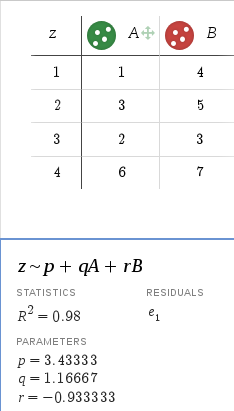# How to fit an equation containing more that one variables?

Hello everyone,

I have an equation that depends on more than one variable. I know that if the dependence is `y=f(x)` then I can use the `TF1` function, e.g.,

`TF1 *fit = new TF1("fit", " + * x + * x* x");`

However, in my case, I want to fit something like `Z = p + q A + r B`, where `A` and `B` are independent variables. As a temporary solution, I am using desmos.com. It fits parameters `p, q,` and `r`. Please let me know how I can do this with ROOT.Thank you,
Divyang.

``````{
const Int_t n = 4;
Double_t z[n] = {1., 2., 3., 4.};
Double_t A[n] = {1., 3., 2., 6.};
Double_t B[n] = {4., 5., 3., 7.};
TGraph2D *g = new TGraph2D(n, A, B, z); // x = A, y = B
TF2 *fit = new TF2("fit", "[p] + [q] * x + [r] * y");
fit->SetParameters(3.5, 1., -1.); // reasonable initial values
g->Fit(fit, "");
// g->SetMarkerStyle(20); g->Draw("PCOL");
}
``````

Hi, this worked!

But what if there are three variables? Should I change it to
`TF3 *fit = new TF3("fit", "[p] + [q] * x + [r] * y + [s]* z");`

I mean what to do if there are three or more independent variables? That is `Z = p + q A + r B + s C`, where `A, B,` and `C` are the independent variables and `p, q, r,` and `s` are the fitting parameters.

There is no “TGraph3D” in ROOT, so you will need to use a different approach, e.g.:

Another possibility would be to create a `TH3?` histogram and fit it with a `TF3` fuction.

Hi Divyang, good to see that you problem is solved … for the moment. So what are going to do when you encounter a problem with 10 independent variables, use a TF10 or TH10 ?

What you are looking for is the solution to a linear regression problem. Implementations in ROOT vary from applying the linear algebra package to using roofit .

-Eddy

1 Like

@Eddy_Offermann Can you recommend any existing ROOT (linear algebra package and/or RooFit) macros?

Have a look at solve_linear.C. Adjust the variable nrVar accordingly.